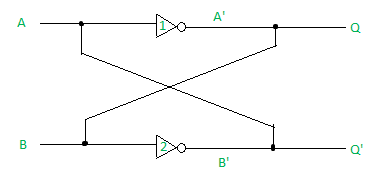Open in App
Not now

# One bit memory cell (or Basic Bistable element)

• Difficulty Level : Basic
• Last Updated : 07 Feb, 2023

A One Bit Memory Cell (also known as a Basic Bistable Element) is a digital circuit that can store a single bit of information. It is a type of sequential circuit that can hold its state until a new input signal is received, causing the state to change. One Bit Memory Cells are used in digital systems as temporary storage elements and are the building blocks of more complex sequential circuits such as flip-flops, latches, and state machines.

### There are two types of One Bit Memory Cells:

1. SR (Set-Reset) Flip-Flops: The SR flip-flop is a type of One Bit Memory Cell that has two inputs, S (Set) and R (Reset). The S input sets the output to 1, while the R input resets the output to 0. When both S and R are at 1, the flip-flop is in an “undefined” state.
2. D (Data) Flip-Flops: The D flip-flop is a type of One Bit Memory Cell that has two inputs: D (Data) and a clock signal. The output of the flip-flop follows the input at the D terminal as long as the clock signal is high. When the clock signal goes low, the output of the flip-flop is stored and held until the next rising edge of the clock.
3. One Bit Memory Cells are widely used in digital systems for various applications, including data storage, control circuits, and state machines. They are often used in combination with other digital circuits to implement sequential circuits, such as memory elements and state machines.

In summary, One Bit Memory Cells (also known as Basic Bistable Elements) are digital circuits that store a single bit of information and hold its value until it is updated by new input signals. There are two types of One Bit Memory Cells: SR (Set-Reset) Flip-Flops and D (Data) Flip-Flops, and they are widely used in digital systems for various applications.

One Bit memory cell is also called Basic Bistable element. It has two cross-coupled inverters, 2 outputs Q and Q’. It is called “Bistable” as the basic bistable element circuit has two stable states logic 0 and logic 1. The following diagram shows the Basic Bistable element:```(A) when A=0,
(i) In inverter1, Q = A'= B= 1
(ii)In inverter2, Q' = B' = A = 0

(B) when A=1,
(i) In inverter1, Q = A'= B= 0
(ii)In inverter2, Q' = B' = A = 1 ```

Some key points:

1. The 2 outputs are always complementary.
2. The circuit has 2 stable states. when Q=1, it is Set state. when Q=0, it is Reset state.
3. The circuit can store 1-bit of digital information and so it is called one-bit memory cell.
4. The one-bit information stored in the circuit is locked or latched in the circuit. This circuit is also called Latch.

One-bit six transistor SRAM memory cell is volatile. Fast switching speed is its main advantage. It needs a constant power supply because of its volatility. The demand for faster and denser memory devices has seen memristors being proposed as replacements for flash memory, Static Random Access Memory (SRAM) and Random Access Memory (RAM). The most salient feature of memristors is its resistive switching ability that can retain resistance states for long periods despite without power supply. The One-bit non-volatile memory cell can be designed using transmission gates and memristor. Memristive devices have high switching speed, low energy consumption, non-volatile and small device size. The non-volatility of memristor used in designs as the information storage element provides retention ability of up to 10 years in the absence of power supply. The transmission gates used ensures that voltage is not lost through other components of the memory cell, thus improves the switching speed by providing a larger potential difference across the memristor. Other advantages of using memristors in memory devices are its long retention period, excellent endurance properties, and good scalability compared to NAND-based flash memory devices. Reference: DIGITAL ELECTRONICS – Atul P. Godse, Mrs. Deepali A. Godse IEEE XPLORE, Digital library – One-bit non-volatile memory cell using memristor and transmission gates.

### Advantages of One Bit Memory Cells:

1. Simplicity: One Bit Memory Cells are simple digital circuits that only require a few transistors to implement. This makes them inexpensive and easy to design.
2. Flexibility: One Bit Memory Cells can be used in a variety of applications, from data storage to control circuits and state machines.
3. Speed: One Bit Memory Cells can operate at high speeds, making them suitable for use in high-speed digital systems.
4. Low Power Consumption: One Bit Memory Cells consume relatively low power, making them ideal for use in battery-powered or low-power applications.

### Disadvantages of One Bit Memory Cells:

1. Limited Storage Capacity: One Bit Memory Cells can only store a single bit of information, making them unsuitable for applications that require more storage capacity.
2. Complexity: When used in complex sequential circuits, One Bit Memory Cells can become more complex and difficult to design, reducing their overall reliability and stability.
3. Cost: As the number of transistors required to implement One Bit Memory Cells increases, the cost of the circuit can become more expensive.

### References:

1. “Digital Design Principles and Practices” by John F. Wakerly.
2. “Digital Systems Design Using VHDL” by Charles H. Roth, Jr. and Lizy K. John.
3. “Digital Circuit Design: An Introduction to the Design of Digital Integrated Circuits” by Mark Burns and Gordon Roberts.
My Personal Notes arrow_drop_up